# 1 Introduction

CancerInSilico provides a streamlined interface for simulating cellular models and gene expression data. The main functions in this package are inSilicoCellModel and inSilicoGeneExpression.

# 2 Running a Cell Simulation

## 2.1 Run Simple Simulation

Every call to inSilicoCellModel must specify the initial number of cells, the run time of the simulation in hours, and the initial density of the cell population. We also set the output increment here to minimize verbosity and the seed to allow for reproducibility.

``````simple_mod <- suppressMessages(inSilicoCellModel(initialNum=30, runTime=72,
density=0.1, outputIncrement=24, randSeed=123))``````
``````##
## time = 0.00
## size = 30
## time = 24.00
## size = 58
## time = 48.00
## size = 107
## time = 72.00
## size = 171``````

## 2.2 Plot CellModel Object

This creates a model object that can be used to generate gene expression data. It is also possible to view the model results directly throught the plotCells function. The plots are colored according to cell phase - cells in interphase are colored gray and cells in mitosis are colored black.

``plotCells(simple_mod, time=0)``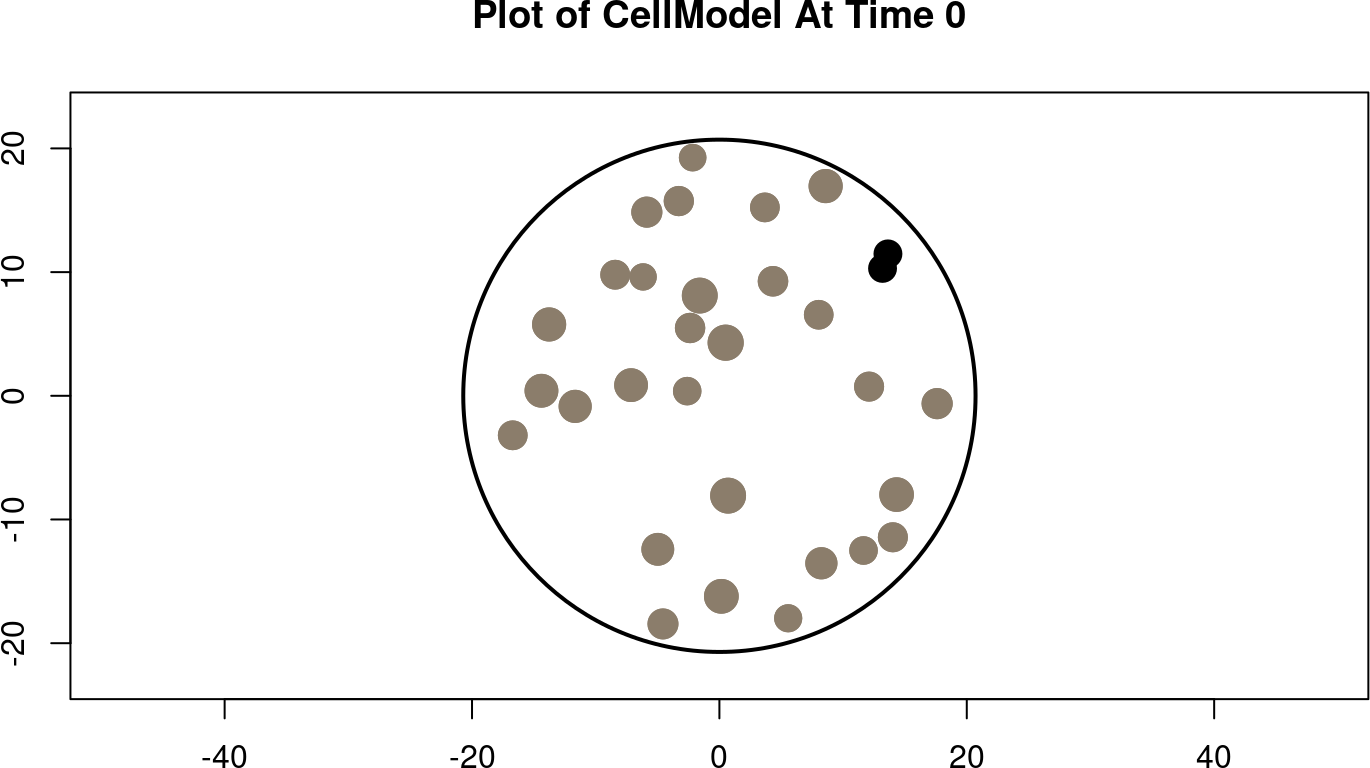``plotCells(simple_mod, time=36)``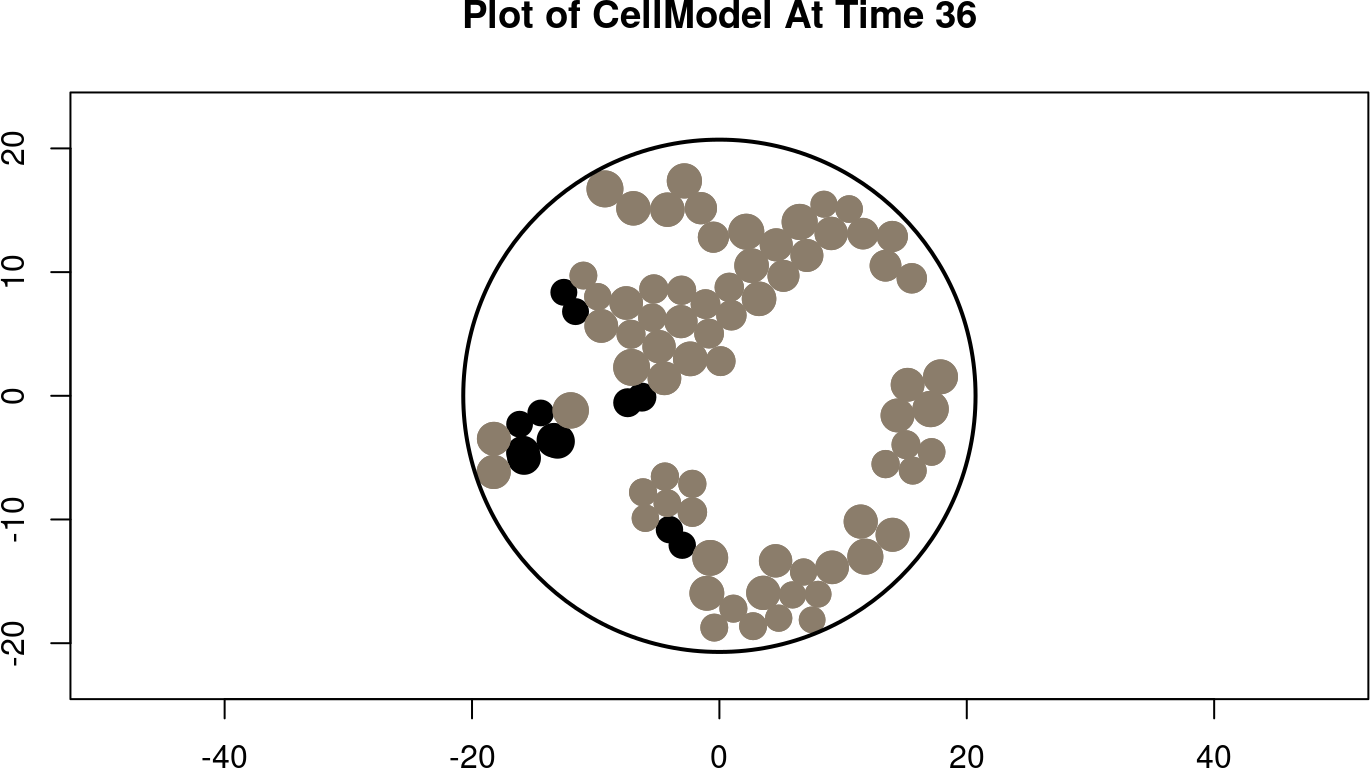``plotCells(simple_mod, time=72)``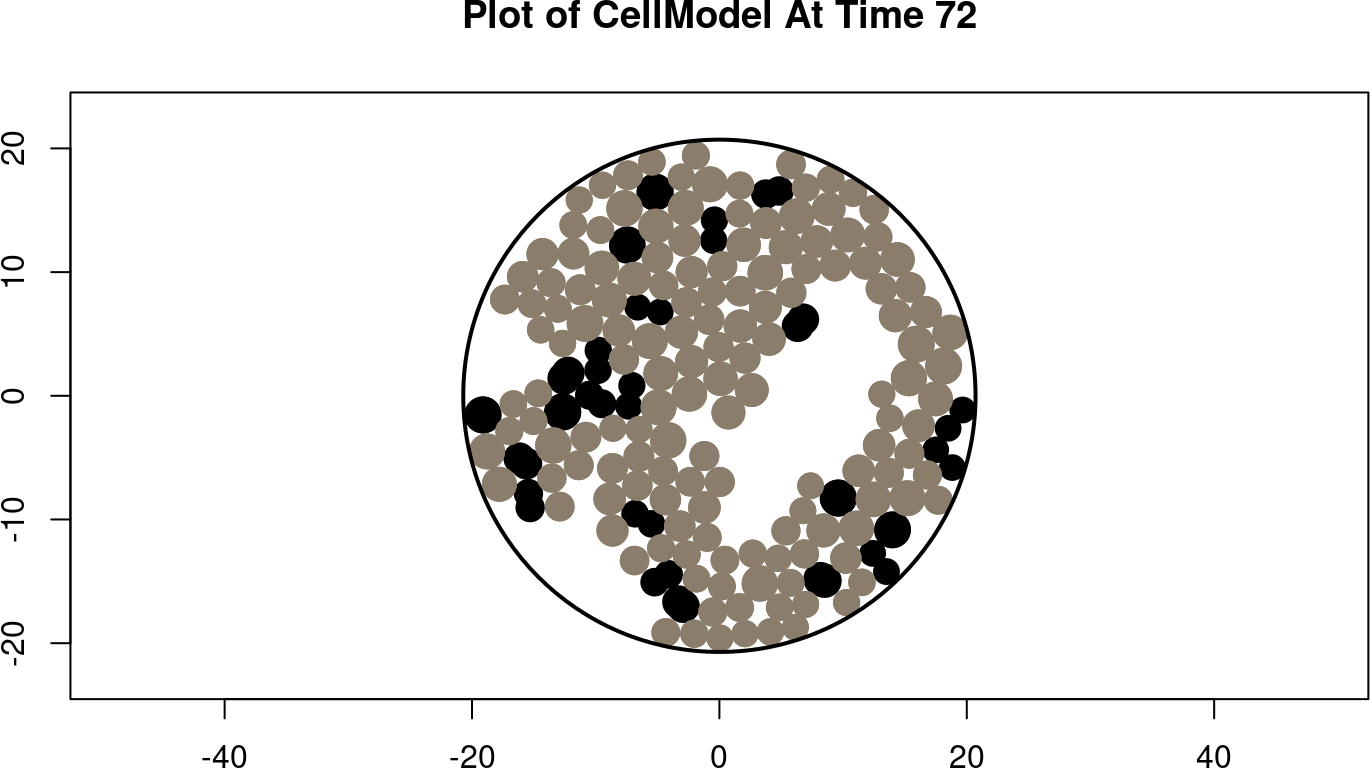## 2.3 Query Cell Information

inSilicoCellModel outputs a CellModel that comes with getter functions to query information about the model. Here we use getNumberOfCells and getDensity to plot the size and density of the population over time.

``````# hours in simulation
times <- 0:simple_mod@runTime

# plot number of cells over time
nCells <- sapply(times, getNumberOfCells, model=simple_mod)
plot(times, nCells, type="l", xlab="hour", ylab="number of cells")``````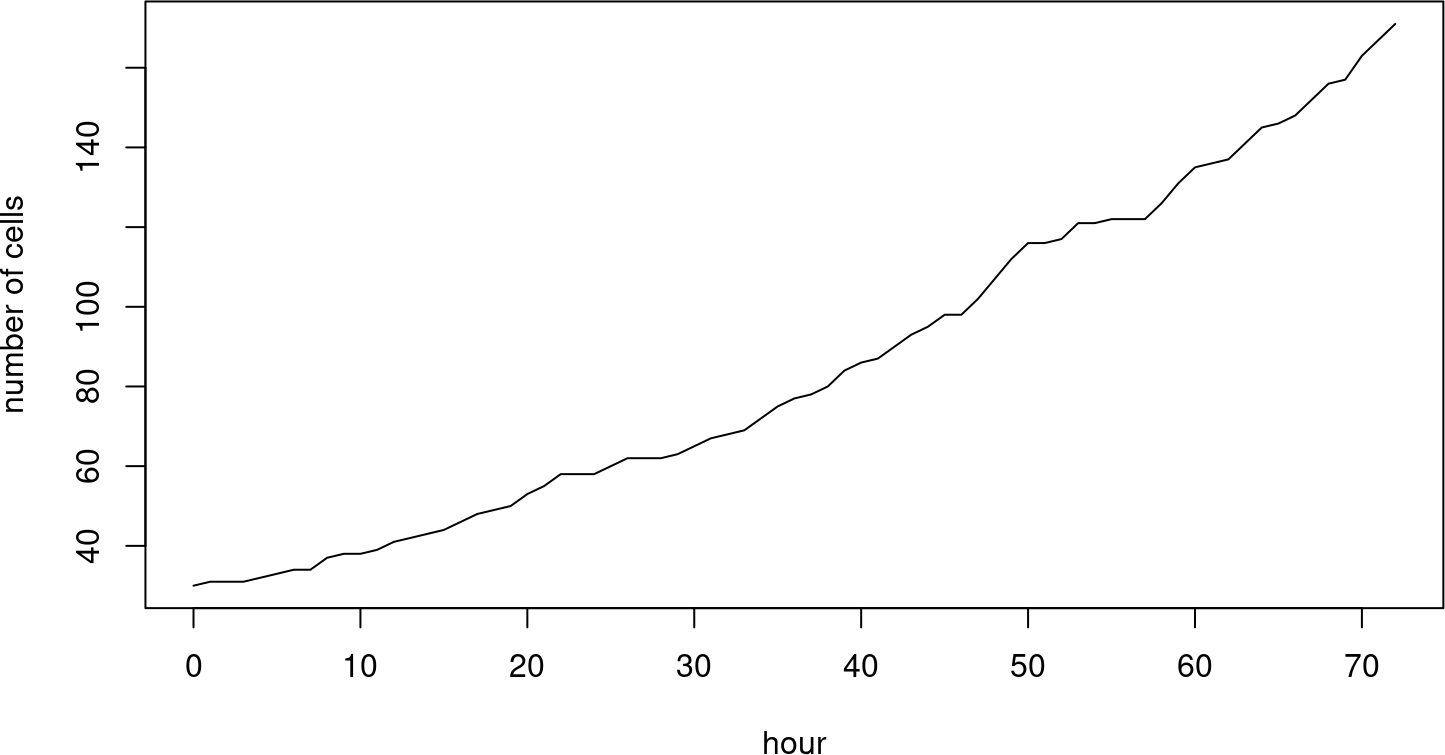``````# plot population density over time
den <- sapply(times, getDensity, model=simple_mod)
plot(times, den, type="l", xlab="hour", ylab="population density")``````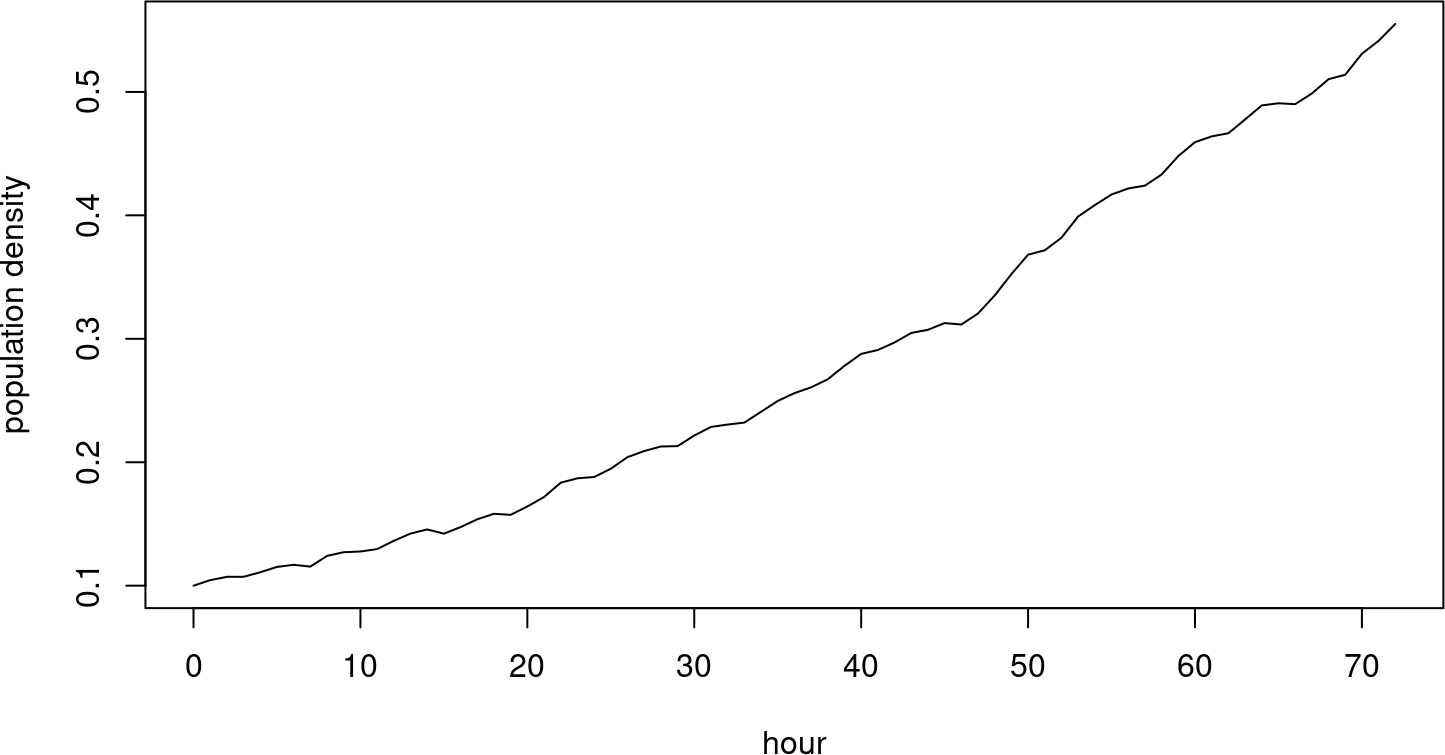# 3 Drugs

inSilicoCellModel supports drugs that function by supressing proliferation. A list of Drug objects can be passed to this function. These objects define a function to calculate the effect of the drug and the time at which the drug is added. The cycleLengthEffect field of the Drug object is a function that takes two parameters, cell type and cell cycle length. It returns the new cell cycle length.

Here we create a drug that cuts proliferation rates in half by doubling the cell cycle length. It is added at 24 hours into the simulation.

``````drug <- new("Drug", name="Drug_A", timeAdded=24,
cycleLengthEffect=function(type, length) length * 2)
drug_mod <- suppressMessages(inSilicoCellModel(initialNum=30, runTime=72,
density=0.1, drugs=c(drug), outputIncrement=24, randSeed=123))``````
``````##
## time = 0.00
## size = 30
## time = 24.00
## size = 58
## time = 48.00
## size = 79
## time = 72.00
## size = 110``````
``````# hours in simulation
times <- 0:simple_mod@runTime

# plot number of cells over time
nCells <- sapply(times, getNumberOfCells, model=simple_mod)
nCells_drug <- sapply(times, getNumberOfCells, model=drug_mod)
plot(times, nCells, type="l", xlab="hour", ylab="number of cells")
lines(times, nCells_drug, type="l", xlab="hour", ylab="number of cells",
col="red")``````